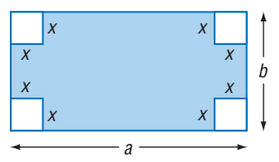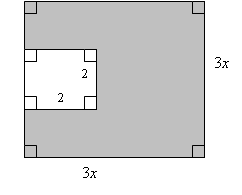### Day 16 - Multiplying Polynomials - 09.09.14

Bell Ringer
1. Write a polynomial to represent the area of the shaded region.1. ab + 4x2

2. -ab + 4x2

3. ab - 4x2

4. 4x2 - ab

5. none of the above

2. Write a polynomial to represent the area of the shaded region.1. (3x)2 - 4

2. 9x2 - 4

3. 5

4. 9x - 5

5. none of the above

3. Write a polynomial to represent the area of the shaded region of the object drawn on the board.

1. πr2 - r2

2. πr2 - 4r2

3. πr2 - 8r2

4. 8r2 - πr2

5. none of the above

4. Simplify the following: -2x2(3x2 - 7x +10)

1. 6x4 + 14x3 +20x2

2. -6x4 + 14x3 - 20x2

3. 6x4 - 14x3 - 20x2

4. -6x4 - 14x3 - 20x2

5. none of the above

5. Simplify the following: (x + 3)(x + 2)

1. x2 + 5

2. x2 + 5x - 6

3. x2 + 5x + 6

4. x2 - 5x + 6

5. none of the above

Review
• Monomials
• Polynomials

Lesson
• Multiplying Polynomials by Monomials
• page 446 #5-11 (odds)
• page 446 #10
• Checkpoint!
• page 446 #15-35 (odds)
• page 446 #36
• Checkpoint!
• page 447 #37-47 (odds)
• page 447 #38 and 48
• Checkpoint!
• Multiplying Polynomials by Polynomials
• page 455 #5-11
• page 455 #10
• Checkpoint!
• page 455 #13-41
• page 455 #38 and 42
• Checkpoint!

Exit Ticket
• Submit the answer to the Exit Ticket given at the end of the block.
Lesson Objective(s)
• How can polynomials by multiplied?
• How is multiplying polynomials related to multiplying regular numbers?

Standard(s)

Mathematical Practice(s)
• #1 - Make sense of problems and persevere in solving them
• #2 - Reason abstractly and quantitatively
• #3 - Construct viable arguments and critique the reasoning of others
• #7 - Look for and make use of structure